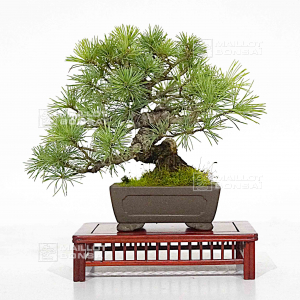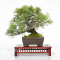##### The Japanese Bonsai specialist
Direct order Contact Help / Services Newsletter# Pinus pentaphylla 170302210

Pinus pentaphyllaref. : 11630

640,00

Available quantity : 1Order

###### Description

Height of the tree only: 160 mm. Width of the branches: 240 mm. Artisanal quality non-enameled pot: 110*80*42 mm. Moyogi style with a superb trunk. Diameter of the trunk: about 30 mm. Width of the nebari: about 60 mm. It was ligatured by master Tomoya Nishikawa in September 2020 and does not have a strong cutting wound or wire mark. It comes from seedlings with very short needles. Requires full sun exposure to keep its beautiful colors. Fertilization from June to October with the Bio-gold fertilizers.

This is a really beautiful mame bonsaï with a sinuous trunk and a formed bark.

It was cultivated at master Shiraichi's nursery for more than 20 years.

It was repoted in pure akadama soil at the end of the 2020 spring. For collector.

Shelf not included.

Photographed in March 2022.

#with 4.4 #bonsai 3.6 #trunk 3.5 #pentaphylla 3.1 #beautiful 2.9 #master 2.6 #about 2.5 #pinus 2.5 #width 2.5 #mini 2.4

Formule
(( ROUND((CHAR_LENGTH(b.article_nom)-CHAR_LENGTH(REPLACE(b.article_nom, 'with', '')))/LENGTH('with')) + ROUND((CHAR_LENGTH(b.article_description)-CHAR_LENGTH(REPLACE(b.article_description, 'with', '')))/LENGTH('with')) ) * 4.4) + (( ROUND((CHAR_LENGTH(b.article_nom)-CHAR_LENGTH(REPLACE(b.article_nom, 'trunk', '')))/LENGTH('trunk')) + ROUND((CHAR_LENGTH(b.article_description)-CHAR_LENGTH(REPLACE(b.article_description, 'trunk', '')))/LENGTH('trunk')) ) * 3.5) + (( ROUND((CHAR_LENGTH(b.article_nom)-CHAR_LENGTH(REPLACE(b.article_nom, 'pentaphylla', '')))/LENGTH('pentaphylla')) + ROUND((CHAR_LENGTH(b.article_description)-CHAR_LENGTH(REPLACE(b.article_description, 'pentaphylla', '')))/LENGTH('pentaphylla')) ) * 3.1) + (( ROUND((CHAR_LENGTH(b.article_nom)-CHAR_LENGTH(REPLACE(b.article_nom, 'beautiful', '')))/LENGTH('beautiful')) + ROUND((CHAR_LENGTH(b.article_description)-CHAR_LENGTH(REPLACE(b.article_description, 'beautiful', '')))/LENGTH('beautiful')) ) * 2.9) + (( ROUND((CHAR_LENGTH(b.article_nom)-CHAR_LENGTH(REPLACE(b.article_nom, 'master', '')))/LENGTH('master')) + ROUND((CHAR_LENGTH(b.article_description)-CHAR_LENGTH(REPLACE(b.article_description, 'master', '')))/LENGTH('master')) ) * 2.6) + (( ROUND((CHAR_LENGTH(b.article_nom)-CHAR_LENGTH(REPLACE(b.article_nom, 'pinus', '')))/LENGTH('pinus')) + ROUND((CHAR_LENGTH(b.article_description)-CHAR_LENGTH(REPLACE(b.article_description, 'pinus', '')))/LENGTH('pinus')) ) * 2.5) + (( ROUND((CHAR_LENGTH(b.article_nom)-CHAR_LENGTH(REPLACE(b.article_nom, 'about', '')))/LENGTH('about')) + ROUND((CHAR_LENGTH(b.article_description)-CHAR_LENGTH(REPLACE(b.article_description, 'about', '')))/LENGTH('about')) ) * 2.5) + (( ROUND((CHAR_LENGTH(b.article_nom)-CHAR_LENGTH(REPLACE(b.article_nom, 'width', '')))/LENGTH('width')) + ROUND((CHAR_LENGTH(b.article_description)-CHAR_LENGTH(REPLACE(b.article_description, 'width', '')))/LENGTH('width')) ) * 2.5) + (( ROUND((CHAR_LENGTH(b.article_nom)-CHAR_LENGTH(REPLACE(b.article_nom, 'from', '')))/LENGTH('from')) + ROUND((CHAR_LENGTH(b.article_description)-CHAR_LENGTH(REPLACE(b.article_description, 'from', '')))/LENGTH('from')) ) * 2.4) + (( ROUND((CHAR_LENGTH(b.article_nom)-CHAR_LENGTH(REPLACE(b.article_nom, 'fertilization', '')))/LENGTH('fertilization')) + ROUND((CHAR_LENGTH(b.article_description)-CHAR_LENGTH(REPLACE(b.article_description, 'fertilization', '')))/LENGTH('fertilization')) ) * 2.3)

## Secure payment## Delivery

Our logistic partners :04 74 55 23 48
Pépinière MAILLOT-BONSAÏ
Le Bois Frazy
01990 RELEVANT - FRANCE
on appointment